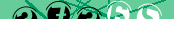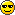# JavaScript: Animating objects over a curved path

The Window.requestAnimationFrame method provides a means for having the browser trigger a function for you at rapid intervals and coordinated with the browser's own refresh cycle. The concept is similar to setTimeout, only faster and smoother.

In previous articles we've explored pure-CSS options for looping animations, but they are limited by the fact that each attribute can only be animated according to specific timing functions.

Using requestAnimationFrame (no jQuery) allows for us to have complete control over both the path and the timing as you can see in the examples below.

## Animating an equation

In the example below there are two animations taking place. Each animation path is defined by a function:

• y = 2 × sin(x);
• y = 2 × cos(x);

The centre of the left border has cartesian coordinates (0,0) and each ball is oscillating between -2/+2 on the y-axis as the x value increases. The animation will repeat every five seconds.

## Animation source code

All you need to reproduce the above animation is the following:

#### HTML markup:

This is where the animation takes place, though elements can also move outside the box if the y-value becomes large enough:

```<div id="field"> <div id="ball"></div> <div id="ball2"></div> </div>```

#### CSS rules

We define a box and place two balls at a starting postion of (0,0):

```<style type="text/css"> #field { position: relative; height: 300px; background-color: lightgreen; } #ball, #ball2 { position: absolute; left: 0; bottom: 50%; width: 1em; height: 1em; border-radius: 0.5em; } #ball { background: red; } #ball2 { background: blue; } </style>```

#### JavaScript code

Every time requestAnimationFrame is called it receives a timestamp parameter which we can use to determine how long the animation has been running.

In this case we've determined that we want the animation to take place over 5 seconds, and use the progress variable to track how much of this has elapsed. This value will be between zero and one - zero placing the balls at the left of the box and one the point at which they reach the right.

To keep things simple the x-axis motion is linear while the y-axis offset is calculated as a function of x. More creative animations could involve projectile or circular motion, and the progress meter itself can be made non-linear or variable.

```<script type="text/javascript"> // Original JavaScript code by Chirp Internet: chirpinternet.eu // Please acknowledge use of this code by including this header. window.requestAnimationFrame = window.requestAnimationFrame || window.mozRequestAnimationFrame || window.webkitRequestAnimationFrame || window.msRequestAnimationFrame; var field = document.getElementById("field"); var ball = document.getElementById("ball"); var ball2 = document.getElementById("ball2"); var maxX = field.clientWidth - ball.offsetWidth; var maxY = field.clientHeight - ball.offsetHeight; var duration = 5; // seconds var gridSize = 50; // pixels var start = null; function step(timestamp) { var progress, x, y, y2; if(start === null) start = timestamp; progress = (timestamp - start) / duration / 1000; // percent x = progress * maxX/gridSize; // x = ƒ(t) y = 2 * Math.sin(x); // y = ƒ(x) y2 = 2 * Math.cos(x); ball.style.left = ball2.style.left = Math.min(maxX, gridSize * x) + "px"; ball.style.bottom = maxY/2 + (gridSize * y) + "px"; ball2.style.bottom = maxY/2 + (gridSize * y2) + "px"; if(progress >= 1) start = null; // reset to start position requestAnimationFrame(step); } requestAnimationFrame(step); </script>```

This code works in the current version of Chrome, Firefox, Safari and Opera, and even Internet Explorer 10. Also in most mobile browsers.

## Circular motion

A small adjustment to the JavaScript code above allows us to propel the ball in a circular or elliptical orbit, as you can see here:

Both the x and y positions now use trigonometric functions based on the elapsed time. We've also inserted a random stretch factor into the code to change the shape of the orbit for each rotation. If you wait long enough you may even see it fly out the side of the box.

```<script type="text/javascript"> // Original JavaScript code by Chirp Internet: chirpinternet.eu // Please acknowledge use of this code by including this header. (function() { window.requestAnimationFrame = window.requestAnimationFrame || window.mozRequestAnimationFrame || window.webkitRequestAnimationFrame || window.msRequestAnimationFrame; var field = document.getElementById("field"); var ball = document.getElementById("ball"); var maxX = field.clientWidth - ball.offsetWidth; var maxY = field.clientHeight - ball.offsetHeight; var duration = 4; // seconds var gridSize = 100; // pixels var start = null; var stretchFactor; function step(timestamp) { var progress, x, y; if(start === null) { start = timestamp; stretchFactor = 1 + (Math.random() * 3); } progress = (timestamp - start) / duration / 1000; // percent x = stretchFactor * Math.sin(progress * 2 * Math.PI); // x = ƒ(t) y = Math.cos(progress * 2 * Math.PI); // y = ƒ(t) ball.style.left = maxX/2 + (gridSize * x) + "px"; ball.style.bottom = maxY/2 + (gridSize * y) + "px"; if(progress >= 1) start = null; // reset to start position requestAnimationFrame(step); } requestAnimationFrame(step); })(); </script>```

By wrapping the code in a self-executing function we prevent the creation of global variables.

For simple animations and hover effects we would still use CSS transforms and @keyframes, saving the more complicated JavaScript option for when animations become too complicated or need to be more interactive.

## References

< JavaScript

#### Send a message to The Art of Web:<- copy the digits from the image into this box

press <Esc> or click outside this box to close

19 December, 2015

Thanks for the helpful refresher. If I may make one suggestion, the expressions

x = 2 × cos(x);
y = 2 × sin(x);

have two reasonable interpretations. It's easy enough to disambiguate, of course, just a small pet peeve of mine.2cos(x) and 2sin(x) would be more explicit.

Yes, it's a messy compromise between the programmers '*' and clean mathematical notation. The first 'x' is actually '&times;', but it's not obvious in the browser. I've italicized the x and y now which might help.top